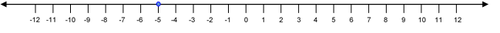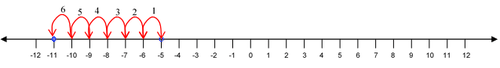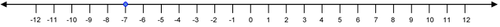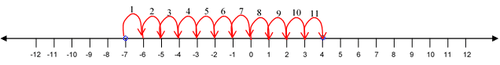•• Contributed by CK12
• CK12Figure $$\PageIndex{1}$$

Smith Middle School has started a school store. Opening day was very busy in the store. Pencils, erasers and pens were sold to sixth, seventh and eighth graders. At the end of the first day, Mallory calculated the total amount of sales and found that the students had raised $87.00. On the second day, Trevor worked in the store. He realized that the school store still owed Mr. Janus$20.00 from the day before, so he took that out of the total sales. Then, he sold $45.00 more dollars in supplies to students. On the third day, Kelly worked in the store and there were three returns for a total of$3.00. Then, Kelly sold $25.00 in supplies. When the students met with Mr. Janus on Wednesday afternoon, they were asked to calculate their total earnings for the week. In this concept, you will learn to add and subtract integers. ### Adding and Subtracting Integers Integers include the set of whole numbers and their opposites: {... -3, -2, -1, 0, 1, 2, 3 …}. As you work with integers you are working with both positive and negative numbers. You work with integers when adding, subtracting, multiplying and dividing. When working with integers and operations, it’s important to keep track of whether the integer is positive or negative. Let's look at an example. Notice that the number increases but in the negative direction. If you think about this on a number line, you can see how your number becomes more negative. First, draw your number line and place your dot on -5 as this is where your problem starts.Figure $$\PageIndex{1}$$ Next, move the blue dot six places to the left (left because it is negative).Figure $$\PageIndex{1}$$ The answer is -11. You can’t always use a number line as your numbers are sometimes just too big for a number line. Let's look at an example. To add two numbers with opposite signs, you are really subtracting and adding the sign that occurs with the larger number. First, find the difference between the two numbers. Next, since 623 is larger and has a negative sign, the answer is negative. The answer is -408. Let's look at another example using subtraction. First, use the subtraction rule in which you know that to subtract b from a is the same as adding the opposite of b to a. In other words, a−b=a+(−b). Next, since both of the numbers are negative, your answer will be larger but in the negative direction. The answer is -656. Let's look at another example. First, add the opposite of −789. Next, use the rules of addition to evaluate. The answer is 843. ### Examples Example $$\PageIndex{1}$$ Earlier, you were given a problem about total earnings at a school store. The workers need to determine their total earnings for the first few days of sales at the school store. Solution First, list all of the sales and debits from the store. Monday: sales =$87.00

Tuesday: sales = $45.00, debits =$20.00

Wednesday: sales = $25.00, debits =$3.00

Next, write an equation so you can find the total sales.

Then, add to find the total earnings.

The total sales is \$134.

Example $$\PageIndex{1}$$

First, find the difference between the two numbers.

Solution

Next, since 563 is larger and has a positive sign, the answer is positive.

Example $$\PageIndex{1}$$

−7+11

Solution

First, draw your number line and place your dot on −7 as this is where your problem starts.[Figure4]

Next, move the blue dot eleven places to the right (right because it is positive).[Figure5]

Example $$\PageIndex{1}$$

45+−19

Solution

First, find the difference between the two numbers.

Next, since 45 is larger and has a positive sign, the answer is positive.

### Review

1. 6+7=_____

2. 5+(−8)=_____

3. 8+(−8)=_____

4. 6+(−10)=_____

5. 8+(−2)=_____

6. 9+(−4)=_____

7. −14+(−7)=_____

8. −12+(−14)=_____

9. −13+(−10)=_____

10. −18+(−30)=_____

Subtract the following integers.

11. −9−5=_____

12. −8−7=_____

13. −12−8=_____

14. 6−9=_____

15. 10−15=_____

16. 18−(−5)=_____

17. 12−(−4)=_____

18. 23−(−9)=_____

19. −5−(−2)=_____

20. −8−(−5)=_____

### Vocabulary

Term Definition
Integer The integers consist of all natural numbers, their opposites, and zero. Integers are numbers in the list ..., -3, -2, -1, 0, 1, 2, 3...
Irrational Number An irrational number is a number that can not be expressed exactly as the quotient of two integers.
Natural Numbers The natural numbers are the counting numbers and consist of all positive, whole numbers. The natural numbers are the numbers in the list 1, 2, 3... and are often referred to as positive integers.
number line A number line is a line on which numbers are marked at intervals. Number lines are often used in mathematics to show mathematical computations.
operation Operations are actions performed on variables, constants, or expressions. Common operations are addition, subtraction, multiplication, and division.
rational number A rational number is a number that can be expressed as the quotient of two integers, with the denominator not equal to zero.
Real Number A real number is a number that can be plotted on a number line. Real numbers include all rational and irrational numbers.